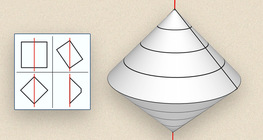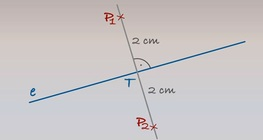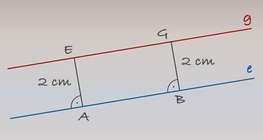Quantity: 0

Total: 0,00

0

# Geometric transformations – reflection

### Related extras#### Solids of revolution (exercise)

An exercise about the generation of solids of revolution.#### Plotting points at a given distance from a line

Line e is given. Plot point P at a distance of 2 cm from line e.#### Light and shadow

By varying the position of the light source, you can examine the shades projected onto...#### Cube of cubes

An exercise about the regular hexahedron built from unit cubes to help deepen your...#### Plotting a parallel line at a given distance

Line e is given. Let’s plot a line parallel to line e 2 cm away.#### Three-dimensional Cartesian coordinate system

3-dimensional Cartesian coordinate system with illustrations and exercises that develop...#### Where exactly?

Learn how to specify a location in a playful manner!#### Plotting a 45° angle

By bisecting a straight angle (180°), we get a right angle (90°). We get a 45° angle by...

Added to your cart.